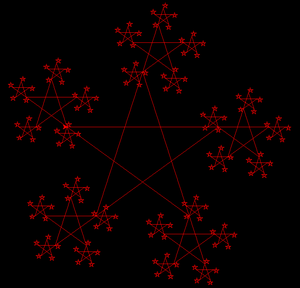Open in App
Not now

# Star fractal printing using Turtle in Python

• Last Updated : 01 Oct, 2020

Prerequisite: Turtle Programming Basics

Fractals are objects that tend to have self-similar structures repeated a finite number of times. The objective of this article is to draw a star fractal where a star structure is drawn on each corner of the star and this process is repeated until the input size reduces to a value of 10. For achieving this star fractal pattern the turtle module is used.

### Methods used

• forward(value): It moves the turtle in forward direction.
• speed(value): It changes the speed of the turtle.
• penup(): Stop drawing.
• pendown(): Start drawing.
• left(value): It moves the turtle towards the left.

### Approach

1. Import turtle.
2. Initialise the turtle.
3. Change the background color.
4. Make a function to draw a star.
5. Call the above function recursively inside the for loop to make the entire start pattern.

Below is the implementation of the above approach.

## Python3

 `# import turtle``import` `turtle`` ` `# initialise turtle instance``stars ``=` `turtle.Turtle()`` ` `# increases the speed of turtle``stars.speed(``10``)`` ` `# to change the background color``stars.getscreen().bgcolor(``"black"``)``stars.color(``"red"``)`` ` `# stop drawing``stars.penup()`` ` `# move the turtle``stars.goto((``-``200``, ``50``))`` ` `# start drawing``stars.pendown()`` ` `# function to draw stars``def` `star(turtle, size):``    ``if` `size <``=` `10``:``        ``return``    ``else``:``        ``for` `i ``in` `range``(``5``):``           ` `            ``# moving turtle forward``            ``turtle.forward(size)``            ``star(turtle, size``/``3``)`` ` `            ``# moving turtle left``            ``turtle.left(``216``)`` ` ` ` `# calling the star function``star(stars, ``360``)``turtle.done()`

Output:My Personal Notes arrow_drop_up